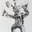Question-and-Answer Resource for the Building Energy Modeling Community
Get s tarted with the Help page

# Energy Plus How to Build a "FaultModel:Fouling:chiller" ?I'm trying to build a "FaultModel:Fouling:chiller" in Energy Plus V8.8. I want to find a result that when chiller condenser fouled, the decrease the "Chiller Condenser Heat Transfer Rate",the increase the "cooling water outlet Temperature",compare to the "Faulty free Model".But there are some weird statement that confuse me. Here are my question:

Question 1 : How to determine the Fouling Factor ? (here is the setting screen - https://ppt.cc/fZN91x) When I am using the "FaultModel:Fouling:chiller" without "Availibility Schedule" and "Severity Schedule", no matter what value I set into the "fouling Factor", the "Chiller Condenser Heat Transfer Rate" is greater than the Faulty free Model . With heat exchangers that have fouling, should the heat exchange be lower? The result is different from the Engineering Reference(P.578) .

Question 2 : How to use the "Severity Schedule" ? (here is the setting screen that severity schedule Value <1 - https://ppt.cc/f4BUPx) (here is the setting screen that severity schedule Value >1 - https://ppt.cc/fTCC4x) When I am using the "FaultModel:Fouling:chiller" with "Severity Schedule" and set the "Fouling Factor" blank. According to the Engineering Reference(P.578) that "The model allows the user to describe the fault using a dynamic fouling factor". I found that when the value in the Schedule is less than 1 , the "Chiller Condenser Heat Transfer Rate" is epual to the Faulty free Model.Then I set the schedule value is greater than 1, the "Chiller Condenser Heat Transfer Rate" is greater than the Faulty free Model. And the "Chiller Condenser Heat Transfer Rate" ratio of the "Fouling" and "the Faulty free" Model is irregular.

Could you do me a favour? Thank you very much

edit retag close merge delete

Sort by » oldest newest most votedFaultModel:Fouling:Chiller reduces the nominal capacity of the chiller. Referring to the Engineering Reference, the fouling factor is applied to the chiller capacity and the chiller COP. If the chiller has adequate capacity for the current load, the "Chiller Evaporator Cooling Rate" will be identical with or without the fouling factor. If the chiller is delivering the same load, but at a reduced COP, then the electric power consumption will be higher and the heat rejection to the condenser will be higher.

The fouling factor is only applied whenever the fault model Availability Schedule is > 0. This much works as stated in the Input Output Reference.

Where it gets tricky (and the Input Output Reference is wrong) is that the Severity Schedule is applied as a divisor to the nominal Fouling Factor, not as a multiplier. Let's assume the Fouling Factor is 0.8 with a Severity Schedule that varies from 1.0 to 1.2. The 1.2 value implies 20% more fouling, so the fouling factor will be 0.8/1.2 = 0.67. A Severity Schedule value of 0.8 would imply 20% less fouling, or 0.8/0.8 = 1.0 = no fouling. As long as you know how the number will be used, then you can decide what to put in the schedule.

Also, if the Severity Schedule is zero, then the nominal Fouling Factor is used. And if the resulting computed fouling factor is >1.0, then 1.0 is used (no fouling).

I've added this to issue #6717 to correct the Input Output Reference description for Severity Schedule which is simply wrong here and in the other fouling fault models.

more